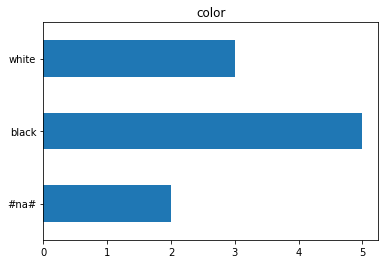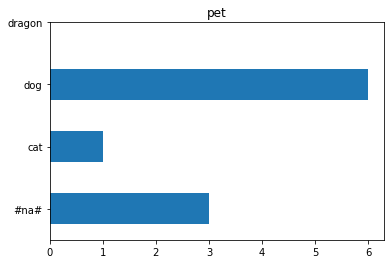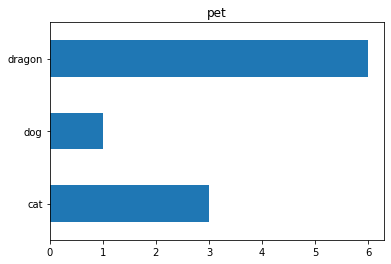# Potential risk of model misinterpretation using TabularPandas in chapter 9

It seems that TabularPandas automatically add a `#na#` class coded as category `zero` to all categorical columns no matter if that column has a missing value or not. Which causes a bit of discrepancy when it comes to mapping column `value_counts` to the related `class`. Especially when implementing partial dependence methadone.

I will use the same method in chapter 9 of the book to create an example case.

``````pet = ['cat', 'dog', 'dragon']
color = ['white', 'black', np.nan]

df = pd.DataFrame({
'pet': [pet[np.random.randint(0,len(pet))] for i in range(10)],
'color': [color[np.random.randint(0,len(color))] for i in range(10)],
'qty': np.random.randint(1, 10, 10)
})

df
``````
pet color qty
0 dragon NaN 5
1 cat NaN 1
2 dragon white 6
3 dragon NaN 9
4 cat black 1
5 cat white 3
6 dragon black 7
7 dog black 4
8 dragon black 9
9 dragon black 3
``````procs = [Categorify, FillMissing]
cont,cat = cont_cat_split(df, dep_var='qty')

to = TabularPandas(df, procs=procs, cat_names=cat,
cont_names=cont, y_names='qty',
splits=RandomSplitter()(range_of(df)))

xs,y = to.xs, to.y
``````

for the cases that the target column includes `NaN` values (like in the book) things sound quite allright. Here for `color` column

``````p = xs['color'].value_counts(sort=False).plot.barh(title='color')
c = to.classes['color']
plt.yticks(range(len(c)), c);
``````But in the case of `pet` column with no missing value, using the same method would suggest that there are `NaN` values.

``````p = xs['pet'].value_counts(sort=False).plot.barh(title='pet')
c = to.classes['pet']
plt.yticks(range(len(c)), c);
``````I suppose in a fairly large dataset with a dozen classes it could become problematic or misleading.
I would suggest:

``````p = xs['pet'].value_counts(sort=False).plot.barh(title='pet')
c = [to.classes['pet'][i] for i in xs['pet'].value_counts(sort=False).index]
plt.yticks(range(len(c)), c);
``````1 Like